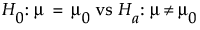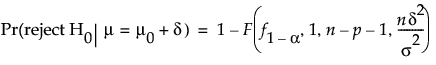Design of Experiments Guide > Prospective Sample Size and Power > One Sample Mean Calculator > Statistical Details for the One Sample Mean Calculator
Publication date: 04/12/2021

## Statistical Details for the One Sample Mean Calculator

The one sample mean calculations are based on the traditional full versus reduced F test for the following hypothesis test:JMP calculates power as follows:where:

α is the significance level.

n is the sample size.

p is the number of extra parameters.

δ is the difference to detect.

f1-α is the (1 - α)th quantile of the F(1, n- p -1) distribution.

F(x, df1, df2, nc) is the cumulative distribution function of the non-central F distribution with degrees of freedom df1 and df2 and non-centrality parameter nc evaluated at x.

Because analytical solutions for δ and n do not exist, numerical solutions are used to solve for them.

For more information about calculations in JMP, see Barker (2011, Section 2.1).

Want more information? Have questions? Get answers in the JMP User Community (community.jmp.com).